# 数据结构之红黑树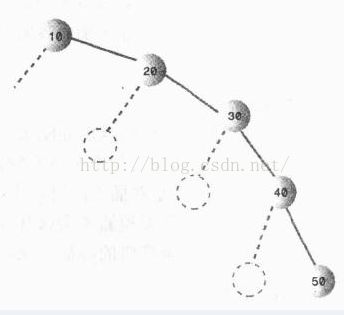### 规则

1. 每一个节点不是黑就是红；
2. 根节点总是黑色的；
3. 红节点的子节点必须是黑色的（红色节点不能连续），反之则不一定成立；
4. 从根节点到叶子节点or空子节点的每条路径 上的黑色节点数目（黑色高度）是相同。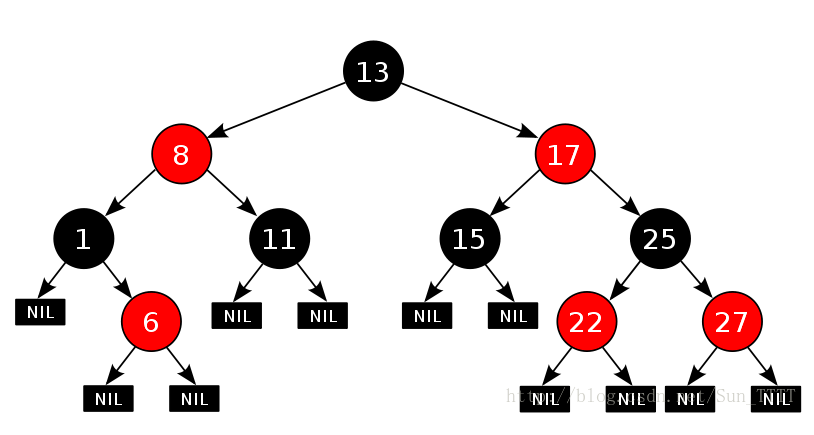### 左旋和右旋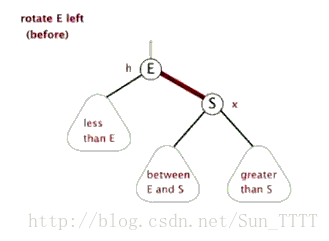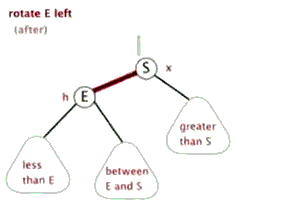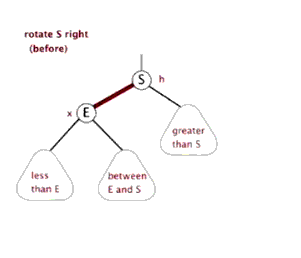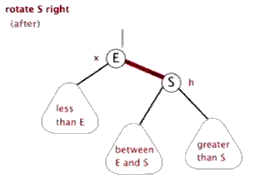### 具体实现

#### 1.红黑树的节点类 RBNode

public class RBNode<T extends Comparable<T>>{
boolean color; //颜色
T key; //关键字(键值)
RBNode<T> left; //左子节点
RBNode<T> right; //右子节点
RBNode<T> parent; //父节点

public RBNode(T key, boolean color, RBNode<T> parent, RBNode<T> left, RBNode<T> right) {
this.key = key;
this.color = color;
this.parent = parent;
this.left = left;
this.right = right;
}

public T getKey() {
return key;
}

public String toString() {
return "" + key + (this.color == RED? "R" : "B");
}
}


#### 2.左旋的具体实现

/*************对红黑树节点x进行左旋操作 ******************/
/*
* 左旋示意图：对节点x进行左旋
*     p                       p
*    /                       /
*   x                      y
*  / \                     / \
* lx  y      ----->   x  ry
*     / \                / \
*   ly  ry            lx   ly
* 左旋做了三件事：
* 1. 将y的左子节点赋给x的右子节点,并将x赋给y左子节点的父节点(y左子节点非空时)
* 2. 将x的父节点p(非空时)赋给y的父节点，同时更新p的子节点为y(左或右)
* 3. 将y的左子节点设为x，将x的父节点设为y
*/
private void leftRotate(RBNode<T> x) {
//1. 将y的左子节点赋给x的右子节点，并将x赋给y左子节点的父节点(y左子节点非空时)
RBNode<T> y = x.right;
x.right = y.left;

if(y.left != null)
y.left.parent = x;

//2. 将x的父节点p(非空时)赋给y的父节点，同时更新p的子节点为y(左或右)
y.parent = x.parent;

if(x.parent == null) {
this.root = y; //如果x的父节点为空，则将y设为父节点
} else {
if(x == x.parent.left) //如果x是左子节点
x.parent.left = y; //则也将y设为左子节点
else
x.parent.right = y;//否则将y设为右子节点
}

//3. 将y的左子节点设为x，将x的父节点设为y
y.left = x;
x.parent = y;
}


#### 3.右旋的具体实现

/*************对红黑树节点y进行右旋操作 ******************/
/*
* 左旋示意图：对节点y进行右旋
*        p                   p
*       /                   /
*      y                   x
*     / \                 / \
*    x  ry   ----->      lx  y
*   / \                     / \
* lx  rx                   rx ry
* 右旋做了三件事：
* 1. 将x的右子节点赋给y的左子节点,并将y赋给x右子节点的父节点(x右子节点非空时)
* 2. 将y的父节点p(非空时)赋给x的父节点，同时更新p的子节点为x(左或右)
* 3. 将x的右子节点设为y，将y的父节点设为x
*/
private void rightRotate(RBNode<T> y) {
//1. 将y的左子节点赋给x的右子节点，并将x赋给y左子节点的父节点(y左子节点非空时)
RBNode<T> x = y.left;
y.left = x.right;

if(x.right != null)
x.right.parent = y;

//2. 将x的父节点p(非空时)赋给y的父节点，同时更新p的子节点为y(左或右)
x.parent = y.parent;

if(y.parent == null) {
this.root = x; //如果x的父节点为空，则将y设为父节点
} else {
if(y == y.parent.right) //如果x是左子节点
y.parent.right = x; //则也将y设为左子节点
else
y.parent.left = x;//否则将y设为右子节点
}

//3. 将y的左子节点设为x，将x的父节点设为y
x.right = y;
y.parent = x;
}


#### 4. 插入操作

/*********************** 向红黑树中插入节点 **********************/
public void insert(T key) {
RBNode<T> node = new RBNode<T>(key, RED, null, null, null);
if(node != null)
insert(node);
}

//将节点插入到红黑树中，这个过程与二叉搜索树是一样的
private void insert(RBNode<T> node) {
RBNode<T> current = null; //表示最后node的父节点
RBNode<T> x = this.root; //用来向下搜索用的

//1. 找到插入的位置
while(x != null) {
current = x;
int cmp = node.key.compareTo(x.key);
if(cmp < 0)
x = x.left;
else
x = x.right;
}
node.parent = current; //找到了位置，将当前current作为node的父节点

//2. 接下来判断node是插在左子节点还是右子节点
if(current != null) {
int cmp = node.key.compareTo(current.key);
if(cmp < 0)
current.left = node;
else
current.right = node;
} else {
this.root = node;
}

//3. 将它重新修整为一颗红黑树
insertFixUp(node);
}


1.插入节点的父节点和其叔叔节点（祖父节点的另一个子节点）均为红色的；

2.插入节点的父节点是红色，叔叔节点是黑色，且插入节点是其父节点的右子节点；

3.插入节点的父节点是红色，叔叔节点是黑色，且插入节点是其父节点的左子节点。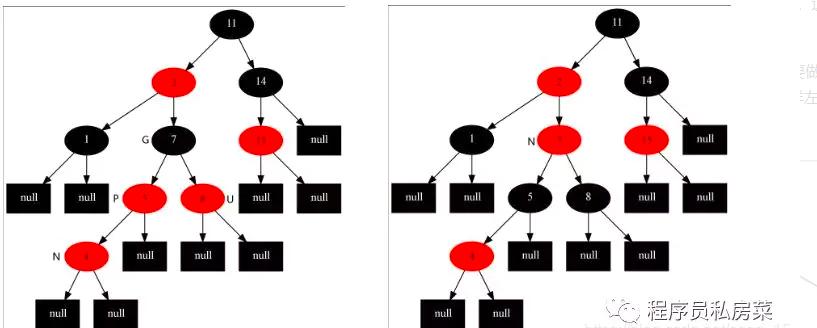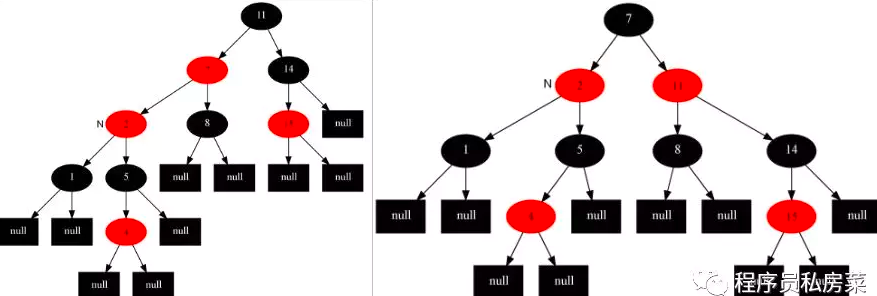private void insertFixUp(RBNode<T> node) {
RBNode<T> parent, gparent; //定义父节点和祖父节点

//需要修整的条件：父节点存在，且父节点的颜色是红色
while(((parent = parentOf(node)) != null) && isRed(parent)) {
gparent = parentOf(parent);//获得祖父节点

//若父节点是祖父节点的左子节点，下面else与其相反
if(parent == gparent.left) {
RBNode<T> uncle = gparent.right; //获得叔叔节点

//case1: 叔叔节点也是红色
if(uncle != null && isRed(uncle)) {
setBlack(parent); //把父节点和叔叔节点涂黑
setBlack(uncle);
setRed(gparent); //把祖父节点涂红
node = gparent; //将位置放到祖父节点处
continue; //继续while，重新判断
}

//case2: 叔叔节点是黑色，且当前节点是右子节点
if(node == parent.right) {
leftRotate(parent); //从父节点处左旋
RBNode<T> tmp = parent; //然后将父节点和自己调换一下，为下面右旋做准备
parent = node;
node = tmp;
}

//case3: 叔叔节点是黑色，且当前节点是左子节点
setBlack(parent);
setRed(gparent);
rightRotate(gparent);
} else { //若父节点是祖父节点的右子节点,与上面的完全相反，本质一样的
RBNode<T> uncle = gparent.left;

//case1: 叔叔节点也是红色
if(uncle != null & isRed(uncle)) {
setBlack(parent);
setBlack(uncle);
setRed(gparent);
node = gparent;
continue;
}

//case2: 叔叔节点是黑色的，且当前节点是左子节点
if(node == parent.left) {
rightRotate(parent);
RBNode<T> tmp = parent;
parent = node;
node = tmp;
}

//case3: 叔叔节点是黑色的，且当前节点是右子节点
setBlack(parent);
setRed(gparent);
leftRotate(gparent);
}
}

//将根节点设置为黑色
setBlack(this.root);
}


package test;

import tree.RBTree;

public class RBTreeTest {

private static final int a[] = {10, 40, 30, 60, 90, 70, 20, 50, 80};
private static final boolean mDebugInsert = true;    // "插入"动作的检测开关(false，关闭；true，打开)
private static final boolean mDebugDelete = true;    // "删除"动作的检测开关(false，关闭；true，打开)

public static void main(String[] args) {
int i, ilen = a.length;
RBTree<Integer> tree = new RBTree<Integer>();

System.out.printf("== 原始数据: ");
for(i=0; i<ilen; i++)
System.out.printf("%d ", a[i]);
System.out.printf("\n");

for(i=0; i<ilen; i++) {
tree.insert(a[i]);
// 设置mDebugInsert=true,测试"添加函数"
if (mDebugInsert) {
System.out.printf("== 添加节点: %d\n", a[i]);
System.out.printf("== 树的详细信息: \n");
tree.print();
System.out.printf("\n");
}
}

System.out.printf("== 前序遍历: ");
tree.preOrder();

System.out.printf("\n== 中序遍历: ");
tree.inOrder();

System.out.printf("\n== 后序遍历: ");
tree.postOrder();
System.out.printf("\n");

System.out.printf("== 最小值: %s\n", tree.minValue());
System.out.printf("== 最大值: %s\n", tree.maxValue());
System.out.printf("== 树的详细信息: \n");
tree.print();
System.out.printf("\n");

// 设置mDebugDelete=true,测试"删除函数"
if (mDebugDelete) {
for(i=0; i<ilen; i++)
{
tree.remove(a[i]);

System.out.printf("== 删除节点: %d\n", a[i]);
System.out.printf("== 树的详细信息: \n");
tree.print();
System.out.printf("\n");
}
}
}

}©️2019 CSDN 皮肤主题: 技术黑板 设计师: CSDN官方博客